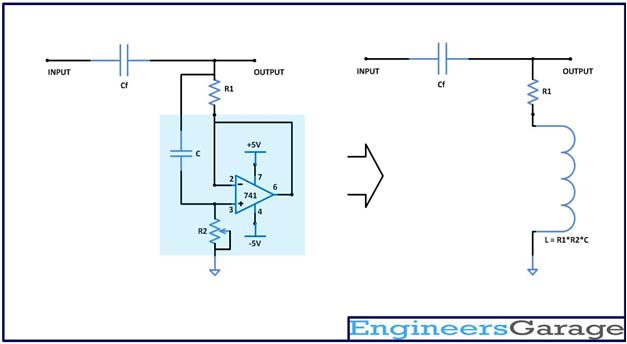﻿ Synthetic Inductor Based High Pass Audio Filter: Circuit Design

# Synthetic Inductor Based High Pass Audio Filter

The inductor coil is one of the fundamental electronic components however it is very less used in the electronic circuits as compared to the other components. The reasons are mainly due to its physical size, sensitivity to the presence of electromagnetic field, unavailability of wide range of inductance values etc. The inductors cannot be included in the Integrated Circuit Chips due to its three dimensional geometry and size. Hence most of the circuit designers try to avoid inductor coils from their circuits and prefer ‘Synthetic Inductor Circuits’ which simulates an inductor using other electronic components.

This article discusses on the design of a Synthetic Inductor circuit and demonstrates its working on a sample application circuit. The Synthetic Inductor can be easily made using a capacitor, Op-amp, a resistor and a variable resistor using which the inductance value can be varied by a large range. When using SMD components the entire circuit will take less space than the actual inductor.
Here the Synthetic Inductor is used to realize a simple high pass filter which can remove the low frequency sounds from a normal audio input. The high frequencies are separated from the low frequency bass-treble before it is fed to normal loudspeakers since they cannot effectively reproduce bass-treble like the woofers doesFig. 1: Synthetic Inductor Based High Pass Audio Filter Circuit on Breadboard

The basic concept of the Synthetic Inductor circuit is to use a capacitor and inverse its properties so that it behaves like an inductor. The advantages of this circuit over the actual inductors are the very low internal resistance, easily varying the inductance value in wide ranges, possible to design high quality filter circuits etc.

The circuit diagram of a Synthetic Inductor circuit is given below;Fig. 2: Circuit Diagram of Synthetic Inductor
Here the property of the capacitor ‘C’ in the above circuit has been inverted with the help of the unity gain op-amp circuit. The value of the inductance depends on the values of the resistors R1, R2 and the capacitor C also. The inductance of the Synthetic inductor circuit is given by the following equation;
L = R1 * R2 * C
The value of the inductance increases as the value of the resistors R1 and R2 increases. The R1 also appears as a series resistor for the inductor which is not desired, since it affects the quality of the circuits like filters in which these inductors are used.To increase the quality the R1 is always selected to be a very small value. The capacitor value as it increases also increases the quality of the inductor circuit and is selected always selected as a fixed valued resistor. The value of the inductance is varied by using a potentiometer as the resistor R2.

The following section discusses the one of the application and the design of a Synthetic Inductor circuit in an audio high frequency filter circuit. This kind of circuit can be found at the speaker output side of the audio devices filters out the low frequency woofer sounds from the entire audio and allow passing only high frequency to the speaker.
The high frequency filter can be realized using a single capacitor and a single inductor connected in series in which the one end of the inductor is grounded, input is fed from the free end of the capacitor and the filtered output is taken from the point where the capacitor and the inductor are connected together in series. The circuit diagram and the equivalent circuit of the Synthetic inductor based High Pass filter is given in the following diagram;Fig. 3: Circuit Diagram and Equivalent Circuit of Synthetic Inductor Based High Pass filter

The circuit contains a capacitor in series ‘Cf’ which forms a High Pass filter with the Synthetic inductor circuit. If the inductance of the Synthetic inductor is say ‘L’, then the pass band of the High Pass filter starts from the frequency given by the following equation;Most of the audio frequencies other than the Bass frequencies appear above an average frequency of 700 Hz and hence the High pass filter is designed for a cut-in frequency of 700 Hz. The series capacitor is selected as 10uF.
F = 700 Hz
Cf = 10 uF
Using the previous equation the required value of the inductor can be calculated as 4.7mH.
The design for a Synthetic inductor circuit for an inductance of 4.7mH is discussed in the following section;
Select a very low value resistor as the R1, and let it be 4.7 ohms. Select a 1uF capacitor as the capacitor ‘C’and using the inductance equation;
L = R1 * R2 * C
The value for the ‘R2’ can be calculated as 1000 and hence use a potentiometer of value 1K.
The audio input is fed from a PC and the filtered audio in this experiment is observed with a normal headset, since the headset speakers are designed for the good quality reproduction of both the high frequency sound and low frequency bass. The complete circuit diagram is given below;Fig. 4: Circuit Diagram of Synthetic Inductor Based High Pass Audio Filter with PC and Headset Connections
Play some music in the PC which has lot of bass like the one used in the demonstration of this circuit and there won’t be any low frequency bass sound from the headset.
Video: